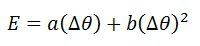# Thermocouple

Definition: The thermocouple is a temperature measuring device. It uses for measuring the temperature at one particular point. In other words, it is a type of sensor used for measuring the temperature in the form of an electric current or the EMF.

The thermocouple consists two wires of different metals which are welded together at the ends. The welded portion was creating the junction where the temperature is used to be measured. The variation in temperature of the wire induces the voltages.

## Working Principle of Thermocouple

The working principle of the thermocouple depends on the three effects.

See back Effect – The See back effect occurs between two different metals. When the heat provides to any one of the metal, the electrons start flowing from hot metal to cold metal. Thus, direct current induces in the circuit.

##In short, it is a phenomenon in which the temperature difference between the two different metals induces the potential differences between them. The See beck effect produces small voltages for per Kelvin of temperature.

Peltier Effect – The Peltier effect is the inverse of the Seebeck effect. The Peltier effect state that the temperature difference can be created between any two different conductors by applying the potential difference between them.

Thompson Effect – The Thompson effect state that when two dissimilar metals join together and if they create two junctions then the voltage induces the entire length of the conductor because of the temperature gradient. The temperature gradient is a physical term which shows the direction and rate of change of temperature at a particular location.

## Construction of Thermocouple

The thermocouple consists two dissimilar metals. These metals are welded together at the junction point. This junction considers as the measuring point. The junction point categorises into three types.

1. Ungrounded Junction – In ungrounded junction, the conductors are entirely isolated from the protective sheath. It is used for high-pressure application works. The major advantage of using such type of junction is that it reduces the effect of the stray magnetic field.
2. Grounded Junction – In such type of junction the metals and protective sheath are welded together. The grounded junction use for measuring the temperature in the corrosive environment. This junction provides resistance to the noise.
3. Exposed Junction – Such type of junction uses in the places where fast response requires. The exposed junction is used for measuring the temperature of the gas.The material uses for making the thermocouple depends on the measuring range of temperature.

### Working of Thermocouple

The circuit of the thermocouple is shown in the figure below. The circuit consists two dissimilar metals. These metals are joined together in such a manner that they are creating two junctions. The metals are bounded to the junction through welding.Let the P and Q are the two junctions of the thermocouples. The T1 and T2 are the temperatures at the junctions. As the temperature of the junctions is different from each other, the EMF generates in the circuit.

If the temperature at the junction becomes equal, the equal and opposite EMF generates in the circuit, and the zero current flows through it. If the temperatures of the junction become unequal, the potential difference induces in the circuit. The magnitude of the EMF induces in the circuit depends on the types of material used for making the thermocouple. The total current flowing through the circuit is measured through the measuring devices.

The EMF induces in the thermocouple circuit is given by the equationWhere Δθ – temperature difference between the hot thermocouple junction and the reference thermocouple junction.
a, b – constants

### Measurement of Thermocouple Output

The output EMF obtained from the thermocouples can be measured through the following methods.

1. Multimeter – It is a simpler method of measuring the output EMF of the thermocouple. The multimeter is connected to the cold junctions of the thermocouple. The deflection of the multimeter pointer is equal to the current flowing through the meter.
2. Potentiometer – The output of the thermocouple can also be measured with the help of the DC potentiometer.
3. Amplifier with Output Devices – The output obtains from the thermocouples is amplified through an amplifier and then feed to the recording or indicating instrument.

The following are the advantages of the thermocouples.

1. The thermocouple is cheaper than the other temperature measuring devices.
2. The thermocouple has the fast response time.
3. It has a wide temperature range.